# Principal Component Analysis (PCA) on astronomical data: interstellar medium (Part I)

There are lots of articles that describe what principal component analysis (PCA) is, when should you use PCA, how to use PCA, and even a thorough walkthrough on the mathematical process behind PCA. What we are going to do in this series (yes, it would be more than one article) is what PCA is widely used for: find the most representative variables that describe the data. In this case, it is the condition of the interstellar medium.

We are going to make an attempt to reproduce the work of Ensor et al. 2017 (Paper I hereafter) that explores the line-of-sight parameters toward stars in the Milky Way. The line-of-sight parameters are equivalent width of diffuse interstellar bands (DIBs), color excess, column density of atomic hydrogen, column density of molecular hydrogen, the fraction of molecular hydrogen, total depletion, and the ratio of the equivalent width of DIB5797 to equivalent width of DIB5780 in 30 line-of-sight.

Diffuse interstellar bands (DIBs) is a set of weak and shallow absorptions originated in the interstellar medium. DIBs are observed toward medium- to high-resolution spectra of astronomical objects located behind interstellar clouds. There are more than 500 identified DIBs in the optical and infrared bands. In Paper 1, the strength of 23 DIB species is used.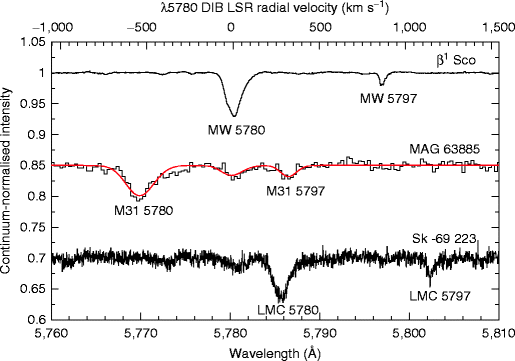Diffuse interstellar bands (DIBs) with central wavelength at around 5780 and 5797 Angstrom observed toward stars in the Milky Way, M31, and LMC (Cordiner 2011).

Notes: In short, those are measurements that describe the environment of interstellar matter toward a star because the space between stars and observer (which means you) is not empty at all. You can treat them as variables that describe you as a person (i.e. name, date of birth, birthplace, height, weight) for more familiar understanding.

All but DIBs equivalent width are provided in Paper 1, so before we proceed to reproduce PCA for all of the variables, we will first demonstrate how PCA performs using only 2 parameters: color excess and hydrogen column densities. The data can be downloaded here and the metadata can also be downloaded here.

Load the data

The cataloged data was transferred from paper 1 into CSV format and it consists of more than 30 x 23 columns since the data not only store the measurements but also the name of the object, the object’s coordinate, additional columns to store uncertainty (as it clear that several of the measurements have asymmetrical uncertainty), the reference from which the measurements are taken, and the source of the spectra.

`import pandas as pddf = pd.read_csv('ensor2017.csv', encoding='latin-1')`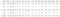Original data, taken from Paper 1.

Calculate the column density of total hydrogen

The power of column density of molecular hydrogen value is stored in a separated column since it is different for each line of sight, therefore the value of it should be calculated first. Paper 1 used this conversion

to calculate the column density of total hydrogen.

`df['n_h2'] = df['n_h2']*10**df['power']df['n_h'] = (df['n_hi']*10**21) + (2*df['n_h2'])`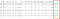Additional column of N(H) or the column density of total hydrogen.

Standardized the data

The paper illustrates how PCA works using two measurements, which were color excess and column density of total hydrogen.

Data standardization is an integral part of performing PCA analysis as the analysis is heavily affected by scaling factor. StandardScaler from sklearn calculates the mean and the variance of the measurements and scales each value into:

where z is the standardized value, x is the original value, u is the mean, and s is the variance. All measurements that will be used in PCA analysis needs to be standardized.

`from sklearn.preprocessing import StandardScalerfeatures = ['ebv','n_h']x = df.loc[:, features].valuesx = StandardScaler().fit_transform(x)`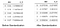The measurements before and after standardization step.

PCA 2D projection

Most PCA practice is aiming to reduce the dimensionality of the data. In this tutorial, we will stick to what the paper has done: keep the dimension as it is.

`import numpy as npfrom sklearn.decomposition import PCApca = PCA(n_components=2)principalComponents = pca.fit_transform(x)principalDf = pd.DataFrame(data = principalComponents,                          columns = ['PC1', 'PC2'])`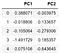Principal component 1 and principal component 2

Find eigenvalues

The eigenvalues of the PCA analysis in Paper 1 were 1.813 for PC1 and 0.187 for PC2 meanwhile in this tutorial we get 1.659 for PC1 and 0.410 for PC2.

`eigenvalues = pca.explained_variance_`Array for PC1 and array for PC2.

Find the variations

Variation is the fraction of total variation in the data for which each PC is responsible (Ensor et al. 2017). The result in the paper was 90.63 for PC 1 and 9.37 for PC2 meanwhile we get 80.16 for PC1 and 19.84 for PC2.

`variation = pca.explained_variance_ratio_`Array for PC1 and array for PC2.

Find the transformation matrix

The transformation matrix is used to obtain transformed data points. The transformed data points are obtained by this notation:

where Y is the transformed data points, A is the transformation matrix, and X is the standardized measurements.

The eigenvector result in the paper is (0.707, 0.707) for PC 1 and (0.707, -0.707) whereas in this tutorial we obtained the same results. Therefore, the transformation matrix is

`component = pca.components_`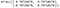The transformation matrix.

Get the transformed data

Based on the previous equation, the transformed data thus:

`principalDf = np.dot(component,x.T)`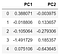Transformed data for PC1 and PC2.

Visualization of standardized original data

Next, we plot the standardized parameters.

`import matplotlib.pyplot as pltfig = plt.figure(figsize = (8,8))ax = fig.add_subplot(1,1,1)ax.set_xlabel('standarized ebv', fontsize = 15)ax.set_ylabel('standarized nh', fontsize = 15)ax.set_ylim(-2,4)ax.set_xlim(-2,4)ax.scatter(x[:,0],x[:,1],s = 50)ax.grid()`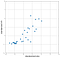Scatter plot of standardized measurements.

Visualize 2D projection

Then we plot the transformed data. Note that we have not taken into account the uncertainty of the measurements involved in this PCA analysis.

`import matplotlib.pyplot as pltfig = plt.figure(figsize = (8,8))ax = fig.add_subplot(1,1,1)ax.set_xlabel('PC1', fontsize = 15)ax.set_ylabel('PC2', fontsize = 15)ax.set_xlim(-3,4.5)ax.set_ylim(-3,3)ax.scatter(principalDf.T[:,0],principalDf.T[:,1],s = 50)ax.grid()`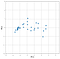Scatter plot of the principal components.

Closing Statement

Next part will be about measuring the diffuse interstellar bands used in Paper 1 so will be able to reconstruct what the paper has done using all the measurements.

An astronomer-in-the-making with interest in data of any fields, astronomical observation, and stellar spectra. Loves coffee and classical music.

## More from Ade N Istiqomah

An astronomer-in-the-making with interest in data of any fields, astronomical observation, and stellar spectra. Loves coffee and classical music.